# RS Aggarwal Solutions for Class 6 Chapter 6 Simplification

## Class 6 RS Aggarwal Chapter 6 – Simplification

RS Aggarwal Solutions for Class 6, Chapter 6 are given here. Students can go through RS Aggarwal Solutions to prepare well for the exam and to score high marks. RS Aggarwal Solutions require a lot of practice to score high marks. RS Aggarwal Solutions help the students to learn the chapter thoroughly.To understand the topics in detail and to gain self-confidence, students are advised to practice the textbook questions.

Students are advised to go through the RS Aggarwal Solutions for Class 6, Chapter 6 in pdf format, solved by the experts at BYJUS in detail. Download PDF of Class 6 Chapter 6 in their respective links.

## Download PDF of RS Aggarwal Solutions for Class 6 Chapter 6 Simplification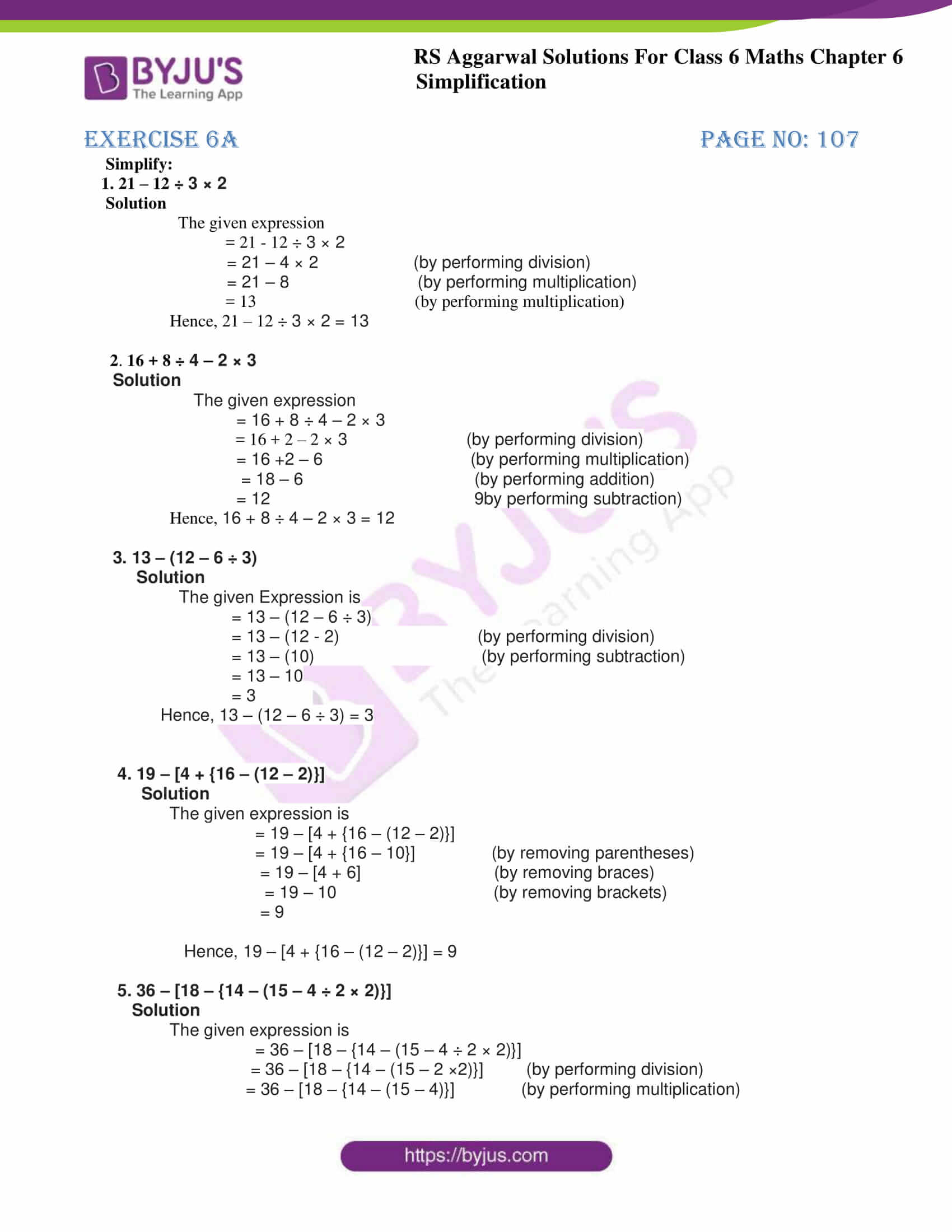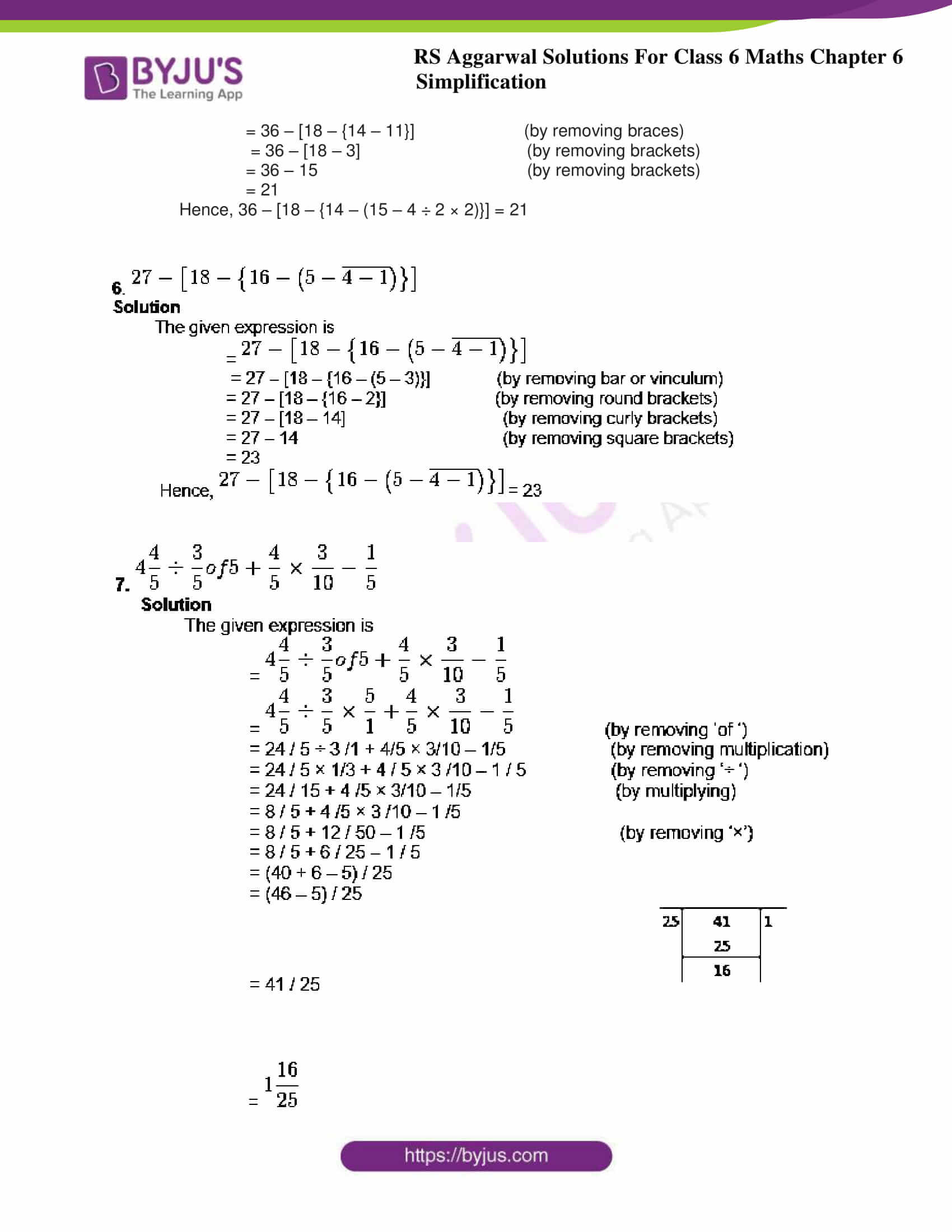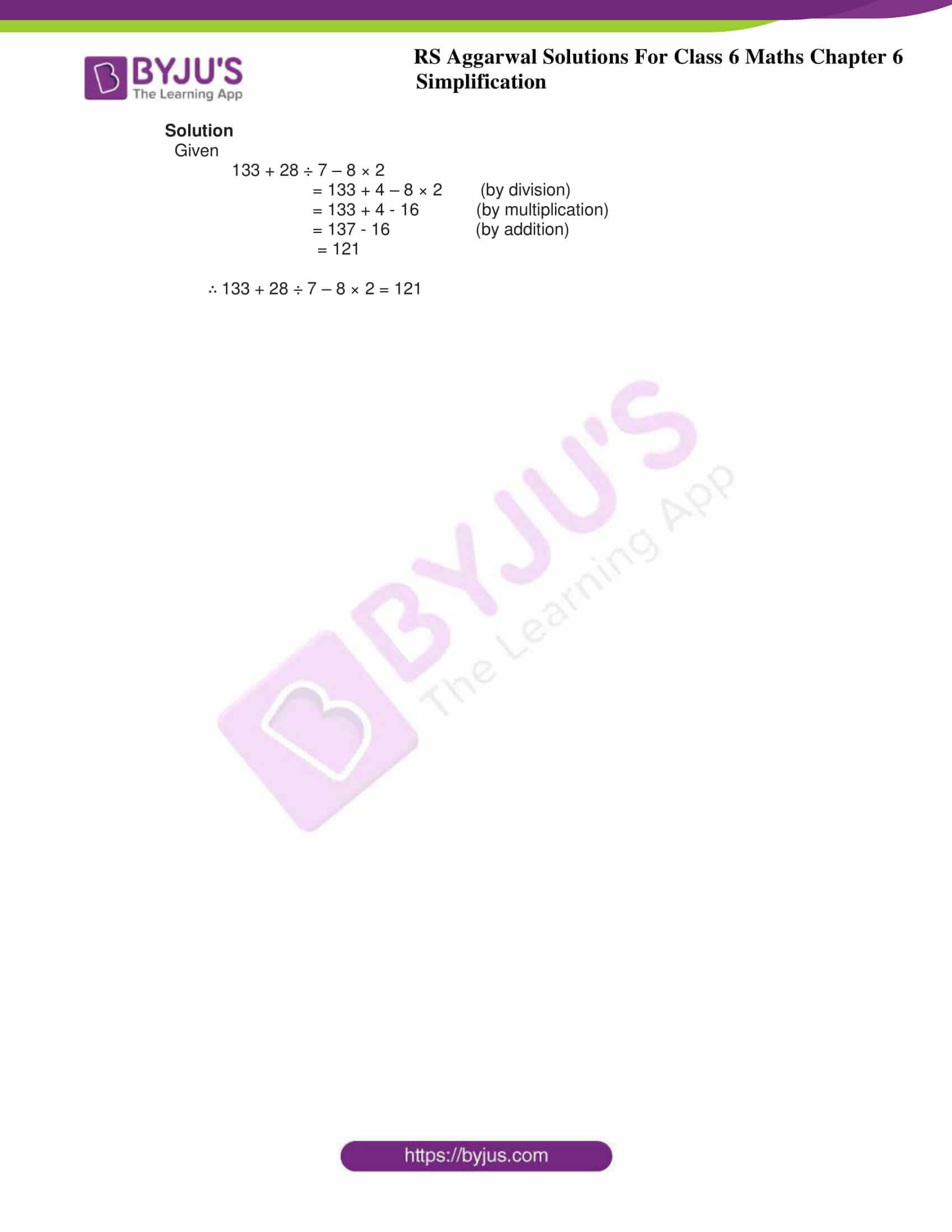## Exercise 6A Page no: 107

Simplify:

1. 21 – 12 ÷ 3 × 2

Solution

The given expression

= 21 – 12 ÷ 3 × 2

= 21 – 4 × 2 (by performing division)

= 21 – 8 (by performing multiplication)

= 13 (by performing multiplication)

Hence, 21 – 12 ÷ 3 × 2 = 13

2. 16 + 8 ÷ 4 – 2 × 3

Solution

The given expression

= 16 + 8 ÷ 4 – 2 × 3

= 16 + 2 – 2 × 3 (by performing division)

= 16 +2 – 6 (by performing multiplication)

= 18 – 6 (by performing addition)

= 12 9by performing subtraction)

Hence, 16 + 8 ÷ 4 – 2 × 3 = 12

3. 13 – (12 – 6 ÷ 3)

Solution

The given Expression is

= 13 – (12 – 6 ÷ 3)

= 13 – (12 – 2) (by performing division)

= 13 – (10) (by performing subtraction)

= 13 – 10

= 3

Hence, 13 – (12 – 6 ÷ 3) = 3

4. 19 – [4 + {16 – (12 – 2)}]

Solution

The given expression is

= 19 – [4 + {16 – (12 – 2)}]

= 19 – [4 + {16 – 10}] (by removing parentheses)

= 19 – [4 + 6] (by removing braces)

= 19 – 10 (by removing brackets)

= 9

Hence, 19 – [4 + {16 – (12 – 2)}] = 9

5. 36 – [18 – {14 – (15 – 4 ÷ 2 × 2)}]

Solution

The given expression is

= 36 – [18 – {14 – (15 – 4 ÷ 2 × 2)}]

= 36 – [18 – {14 – (15 – 2 ×2)}] (by performing division)

= 36 – [18 – {14 – (15 – 4)}] (by performing multiplication)

= 36 – [18 – {14 – 11}] (by removing braces)

= 36 – [18 – 3] (by removing brackets)

= 36 – 15 (by removing brackets)

= 21

Hence, 36 – [18 – {14 – (15 – 4 ÷ 2 × 2)}] = 21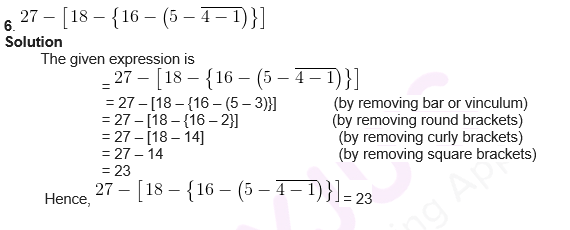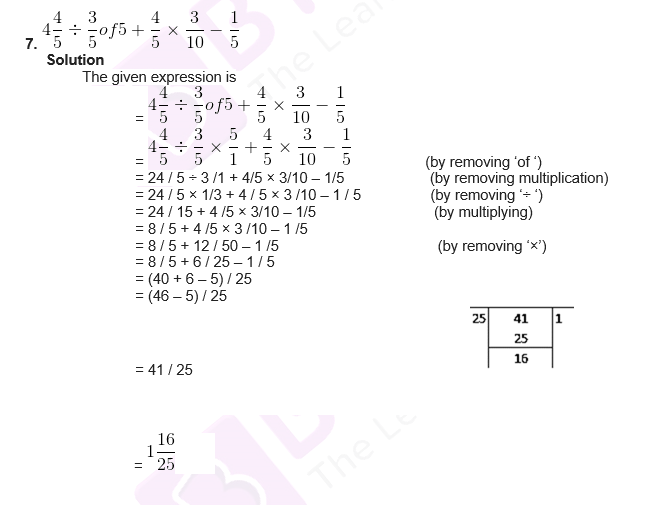## Exercise 6B PAGE NO: 108

OBJECTIVE QUESTIONS

1. 8 + 4 ÷ 2 × 5 = ?

(a) 30 (b) 50 (c) 18 (d) none of these

Solution

Given

8 + 4 ÷ 2 × 5

= 8 + 2 × 5 (by dividing)

= 8 + 10 (by multiplying)

= 18

∴ 8 + 4 ÷ 2 × 5 = 18

2. 54 ÷ 3 of 6 + 9 = ?

(a) 117 (b) 12 (c) 6 / 5 (d) none of these

Solution

Given

54 ÷ 3 of 6 + 9

= 54 ÷ (3 × 6) + 9

= 54 ÷ (18) + 9 (by multiplying)

= 54 ÷ 18 + 9

= 3 + 9 (by dividing)

= 12

∴ 54 ÷ 3 of 6 + 9 = 12

3. 13 – (12 – 6 ÷ 3) = ?

(a) 11 (b) 3 (c) 7 / 3 (d) none of these

Solution

Given

13 – (12 – 6 ÷ 3)

= 13 – (12 – 2) (by dividing)

= 13 – 10 (by subtracting)

= 3

∴ 13 – (12 – 6 ÷ 3) = 3

4. 1001 ÷ 11 of 13 = ?

(a) 7 (b) 1183 (c) 847 (d) none of these

Solution

Given

1001 ÷ 11 of 13

= 1001 ÷ 11 × 13

= 1001 ÷ 143

= 7

∴ 1001 ÷ 11 of 13 = 7

5. 133 + 28 ÷ 7 – 8 × 2 = ?

(a) 7 (b) 121 (c) 30 (d) none of these

Solution

Given

133 + 28 ÷ 7 – 8 × 2

= 133 + 4 – 8 × 2 (by division)

= 133 + 4 – 16 (by multiplication)

= 137 – 16 (by addition)

= 121

∴ 133 + 28 ÷ 7 – 8 × 2 = 121

### RS Aggarwal Solutions for Class 6 Chapter 6 Simplification

Chapter 6 – Simplification includes 2 exercises. RS Aggarwal Solutions for each question in every exercise are solved in detail. Let’s have a glance at the topics mentioned in this chapter

• Use of Brackets
• Square Root

### Chapter Brief of RS Aggarwal Solutions for Class 6 Chapter 6 Simplification

A numerical expression consisting of numbers with two or more of the fundamental operations. We perform these operations in the following order:

Division

Multiplication

Subtraction

There are four kinds of brackets.

Bar, or Vinculum

Round brackets, or small brackets, or Parentheses

Curly brackets, or braces

Square brackets, or Big brackets

The order of simplifying the brackets is:

Bar first

Parentheses next

Braces next

Square brackets in the last